Yoshihara, H., and Kondo, H. (2013). "Effect of specimen configuration and lamination construction on the measurement of the in-plane shear modulus of plywood obtained by the asymmetric four-point bending test," BioRes. 8(3), 3858-3868.

#### Abstract

In this study, the in-plane shear modulus of 3-, 5-, and 7-ply lauan wood (Shorea sp.) was measured by conducting an asymmetric four-point bending (AFPB) test with various specimen depth/length ratios and subsequently performing a flexural vibration (FV) test and finite element analysis (FEA). The results obtained from the experiment and the FEA revealed that the in-plane shear modulus was dependent on the depth/length ratio of the specimen. The dependence of the in-plane shear modulus obtained from the AFPB test was more significant than that obtained from the FV test. Additionally, the in-plane shear modulus values obtained from the AFPB test varied more significantly than those obtained from the FV test. In determining the in-plane shear modulus of plywood, the FV test was therefore superior to the AFPB test.

Effect of Specimen Configuration and Lamination Construction on the Measurement of the In-plane Shear Modulus of Plywood Obtained by the Asymmetric Four-point Bending Test

Hiroshi Yoshihara* and Hiroki Kondo

In this study, the in-plane shear modulus of 3-, 5-, and 7-ply lauan wood (Shorea sp.) was measured by conducting an asymmetric four-point bending (AFPB) test with various specimen depth/length ratios and subsequently performing a flexural vibration (FV) test and finite element analysis (FEA). The results obtained from the experiment and the FEA revealed that the in-plane shear modulus was dependent on the depth/length ratio of the specimen. The dependence of the in-plane shear modulus obtained from the AFPB test was more significant than that obtained from the FV test. Additionally, the in-plane shear modulus values obtained from the AFPB test varied more significantly than those obtained from the FV test. In determining the in-plane shear modulus of plywood, the FV test was therefore superior to the AFPB test.

Keywords: Asymmetric four-point bending test; Finite element analysis; Flexural vibration test; In-plane shear modulus; Plywood

Contact information: Faculty of Science and Engineering, Shimane University, Nishikawazu-cho 1060, Matsue, Shimane 690-8504, Japan; *Corresponding author: yosihara@riko.shimane-u.ac.jp

INTRODUCTION

Plywood is one of the principal wood products used in the construction of shear walls. Therefore, obtaining reliable measurements of the in-plane shear properties, including the in-plane shear modulus, is necessary to ensure that construction procedures are effective. Various test methods have been examined to measure the shear modulus of solid wood and wood-based materials (Yoshihara 2006). Yoshihara (2009a, 2012) investigated asymmetric four-point bending (AFPB) and flexural vibration (FV) tests for measuring the in-plane shear modulus of plywood. The FV test is advantageous because it can be conducted nondestructively. In the FV test, however, the shear modulus cannot be determined without using the Young’s modulus value, so multiple vibration tests are required to determine these moduli. In the AFPB test, the in-plane shear modulus can be simply measured using a single bending test based on elementary beam theory, although an apparatus for measuring the shear strain, such as a strain gage, is required. Recently, there have been several examples of the use of AFPB tests to measure the shear properties of solid wood and laminated veneer lumber (LVL) because of its simplicity (Yoshihara and Suzuki 2005; Morita et al. 2006; Yoshihara 2009b; Chida et al. 2012).

In a previous study, the in-plane shear modulus of 5-ply plywood was investigated using FV and AFPB tests by varying the depth/length ratio of the specimen from 0.06 to 0.08 (Yoshihara 2009a). In this narrow range of depth/length ratios, the effect of the specimen configuration was not significant. In later studies (Yoshihara 2009b, 2010, 2013a), however, the variation of specimen configuration markedly affected the accuracy of the elastic modulus measurements of plywood. Additionally, the variation was enhanced by the lamination construction of plywood. These effects were also observed in the in-plane shear modulus values of plywood measured by FV tests (Yoshihara 2012). To measure the in-plane shear modulus accurately by the FV tests, the length of the specimen must be less than 10 times the depth (Yoshihara 2012). The restriction of specimen configuration may also be applicable to the AFPB test.

In this work, AFPB tests were performed on lauan 3-, 5-, and 7-ply wood specimens with various depth/length ratios, and in-plane shear modulus values were obtained. The validity of the method was examined by comparing its results with the results obtained from the flexural vibration (FV) tests and the finite element (FE) calculations.

Finite Element Analysis (FEA)

Figure 1 shows a diagram of a plywood panel. The longitudinal and tangential directions of the surface veneer coincide with the length and width directions of the plywood. As shown in Fig. 1a, the length, width, and thickness directions of the plywood panel are defined as the xy, and z directions, respectively. A specimen with a length direction that is coincident with the longitudinal direction of the surface veneer is defined as an L-type specimen, whereas a specimen with a length direction that is coincident with the tangential direction of the surface veneer is defined as a T-type specimen. As shown in Fig. 1b, the thicknesses of the surface veneer and the veneer adjacent to the surface veneer are defined as ta and tb, respectively. The length, width, and thickness directions of a beam specimen cut from the plywood panel were defined as the XY, and Z directions, respectively. Therefore, the X-axes of the L- and T-type specimens coincided with the x- and y-axes of the plywood panel, respectively. These definitions were similar to those used in a previous study (Yoshihara 2012).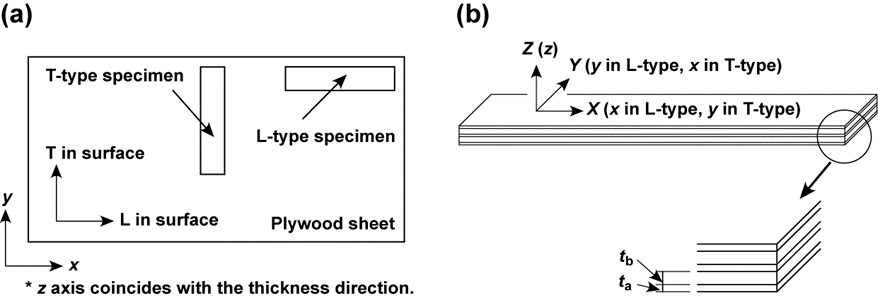Fig. 1. (a) Diagram of the plywood panel and types of specimens; (b) definitions of XY, and Z axes

Three-dimensional (3-D) FEA was performed prior to the FV and AFPB tests using the FEA program ANSYS 12.0 (Ansys 2009). Figure 2 presents a diagram of the AFPB test and FE mesh of the model. Similar to a previous study (Yoshihara 2012), the following four different compositions were simulated: 3-ply, 5-ply, 7-ply, and solid models. Details of the mesh are provided in Fig. 2b and Fig. 2c. The model consisted of eight noded brick elements. The solid model was assumed to be homogeneous, and the elastic constants were obtained using classical lamination theory (Hearmon 1948; Takami 1964; Adams et al. 2003; Yoshihara 2012). The model had a length of 320 mm. The depth H was varied from 10 to 60 mm in 10-mm increments. The breadth of the model T, which corresponded to the total thickness of the plywood panel, was given values of 6, 9, and 12 mm, and the thickness of each veneer was determined as indicated in Table 1. The total thicknesses of the surface veneer and the veneer adjacent to the surface veneer were defined as Ta and Tb, respectively. In the FEA, the ratio Tb/Ta was always constant and equal to 1.5 in these compositions. Table 2 lists the derived elastic constants of lauan (Shorea sp.) for the veneer and the solid model. The elastic constants of the veneer in Table 2 were acquired from data published in the Wood Industry Handbook (Forestry and Forest Products Research Institute 2004). The elastic constants for the solid model were calculated using classical lamination theory. In addition to the lamination construction of the veneers, the glue lines influenced the FEA result. Nairn (2007) conducted the FEA of plywood considering the glue lines, which were simulated using specific elements. Nevertheless, the analysis that considered glue line interfaces in wood was very complex, and therefore was not inlcuded in the objective of this study. Future studies should address the influence of the glue line, although the glue lines were not modeled in the FEA in this study.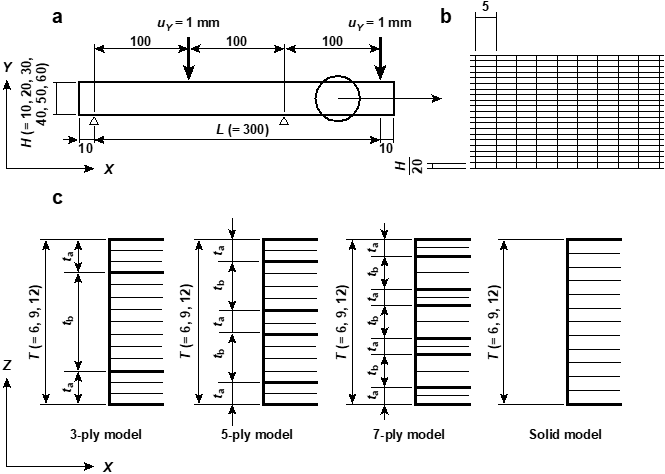Fig. 2. Diagram of the asymmetric four-point bending (AFPB) test and finite element (FE) model for the plywood beams in the XY and XZ planes; unit = mm

Table 1. Construction of the Plywood Models Used in the Finite Element Analyses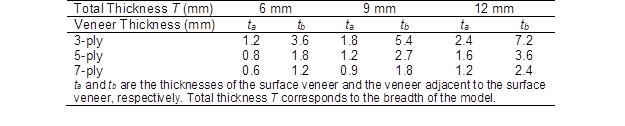Table 2. Elastic Properties Used for the Finite Element Analysis Calculations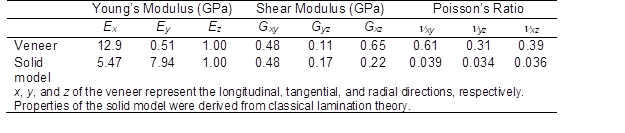As illustrated in Fig. 2a, the nodes corresponding to the locations at X = 10 and 210 mm on the bottom surface were constrained, and a vertical displacement (uY) of 1 mm was applied downward to the nodes corresponding to the locations at X = 110 and 310 mm on the top surface. Therefore, the span length L was 300 mm. The total load P, which corresponds to the applied load in the AFPB test, was obtained from the nodal forces at the loading points, whereas the shear strain of the node at the neutral axis of the mid-length of the surface element XYwas used for the analysis. The in-plane shear modulus GXY was determined by substituting P and XY into the following equation, which was derived from elementary beam theory (Yoshihara and Suzuki 2005; Yoshihara 2009a):(1)

According to lamination theory, the value of GXY should be 0.48 GPa for both models, which corresponds to Gxy in Table 2, independent of the depth/span ratio H/L.

Similar to a previous study (Yoshihara 2012), modal analyses were conducted and the resonance frequencies from the 1st to 4th flexural vibration modes were extracted; Eand the shear modulus GXY were determined from the following equations:(2)

where n is the mode number, and  and  are derived using(3)

where I is the secondary moment of inertia, A is the cross-sectional area,  is the density, and s is Timoshenko’s shear factor, which is 1.2 for a specimen with a rectangular cross-section. When the resonance frequency for the nth flexural vibration mode is defined as fnkn is derived using(4)

The GXY values corresponding to each vibration mode were calculated by altering the value of EX, and the coefficient of variation (COV) among the GXY values was determined. The EXvalue that generates the minimum COV among the GXY values and the mean value of GXY can be regarded as the most feasible. Approximation solutions were obtained also using Mathematica 6.

EXPERIMENTAL

Specimens

Similar to a previous study (Yoshihara 2012), 3-, 5-, and 7-ply samples of lauan wood (Shorea sp.), which were 6, 9, and 12 mm thick, respectively, were investigated. The samples were fabricated by Ueno Mokuzai Kogyo Co. (Himeji, Japan) and had initial lengths and widths of 1820 and 910 mm, respectively. Table 3 lists the thicknesses of the veneers and the densities of each plywood panel. Although the composition of the actual plywood samples was different from the samples adopted for the FE models, the effect of the plywood composition and specimen configuration could still be evaluated. The plywood samples were stored in a room at a constant temperature of 20 C and a relative humidity of 65% before and during the test, and the samples were confirmed to be in an air-dried condition. These conditions were maintained throughout the tests. The equilibrium moisture content (EMC) was approximately 12%.

Table 3. Thickness of the Veneer of the Plywood Used for the Actual Tests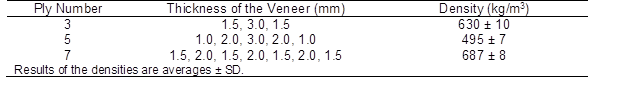The L-type specimens had initial length and depth dimensions of 320 (X = x) 60 (Y = y) mm2, whereas the T-type specimens had initial length and depth dimensions of 320 (X = y) 60 (Y = x) mm2. Ten specimens were prepared of each type, and the densities listed in Table 3 were measured using these twenty specimens with the initial dimensions.

Flexural Vibration (FV) Tests

A previous study suggests that by using a specimen with a depth/length ratio ranging from 0.1 to 0.2, the in-plane shear modulus of plywood can be obtained accurately from FV tests while reducing the effect of the specimen’s configuration (Yoshihara 2012). Therefore, when conducting FV tests using the specimen with the initial configuration, it was thought that the in-plane shear modulus could be measured accurately. To examine the validity of the AFPB test, the FV test was performed before conducting the AFPB tests.

A specimen with the initial configuration was suspended by threads at the nodal positions of the free-free resonance vibration mode fn and excited in the depth direction of the specimen (Y) with a hammer. The resonance frequencies of the 1st to 4th mode were measured and analyzed using a fast Fourier transform (FFT) analysis program. Similar to the FEA method, the EXand GXY values were calculated using Mathematica 6 (2007). This method was similar to that adopted in previous works (Yoshihara 2012). The GXY value obtained from the FV tests was compared with that obtained from the AFPB tests, the details of which are described below.

Asymmetric Four-point Bending (AFPB) Tests

AFPB tests were conducted after performing the FV tests. A biaxial strain gage (gage length = 2 mm; FCA-2-11, Tokyo Sokki Kenkyujo, Tokyo, Japan) was bonded at the center of the surface veneer plane using cyano-acrylate adhesive to measure the shear strain XY. As shown in Fig. 3a and Fig. 3b, the gage axes were oriented at 45 with respect to the X direction. In using the strain gage, there was a concern that the adhesive would reinforce the bonded region of the specimen, which is less stiff (Yoshihara 2013b). Nevertheless, it was difficult to measure the shear strain using a different technique, such as digital image correlation (DIC), which does not require adhesive but does require a special apparatus. Further research should be conducted on the shear strain measurement technique.

As shown in Fig. 3c, the specimen was eccentrically supported at two trisected points, and the loads were applied at the remaining two points. The total span length (L), defined as the distance between the left supporting point and the right loading point, was 300 mm. The specimen was carefully loaded until the shear strain reached 0.0005 to prevent the specimen from inducing the material nonlinearity, then it was immediately unloaded. To reduce the effect of the loading speed, the crosshead speed was varied (as indicated in Table 4). The total testing time was approximately 3 min.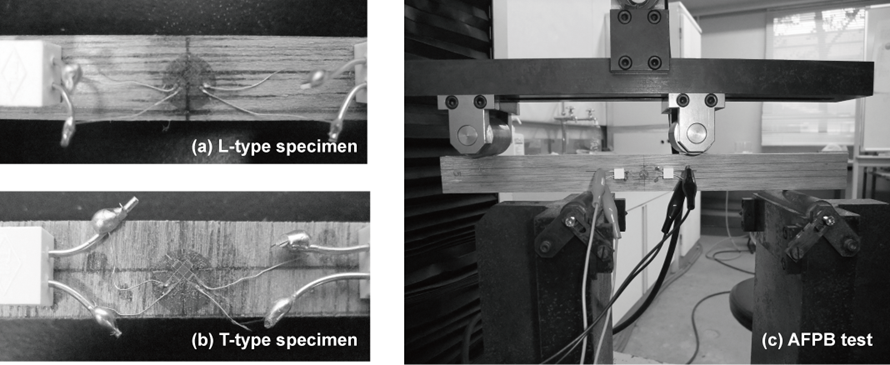Fig. 3. Photographs of the (a) and (b) biaxial strain gage arrangement for the L-type and T-type specimens, respectively, and (c) the asymmetric four-point bending (AFPB) test

Table 4. Crosshead Speed Corresponding to the Specimen’s Depth H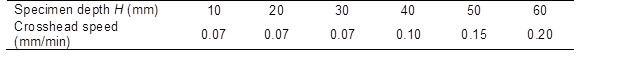By substituting the initial slope of the relationship between the load (P) and shear strain (XY) in Eq. 1, the in-plane shear modulus GXY was obtained. After conducting the AFPB tests, the depth of the specimen was reduced, and the succeeding series of AFPB tests was conducted using the specimens with reduced depth. The depth (H) of the specimens was reduced from 60 to 10 mm in intervals of 10 mm. The GXY values corresponding to the depth/span ratios (H/L) were obtained and compared with the values obtained from the FV tests.

RESULTS AND DISCUSSION

Finite Element Analyses

Figure 4 demonstrates the dependence of the in-plane shear modulus (GXY) on the H/L ratio, as determined by FEA.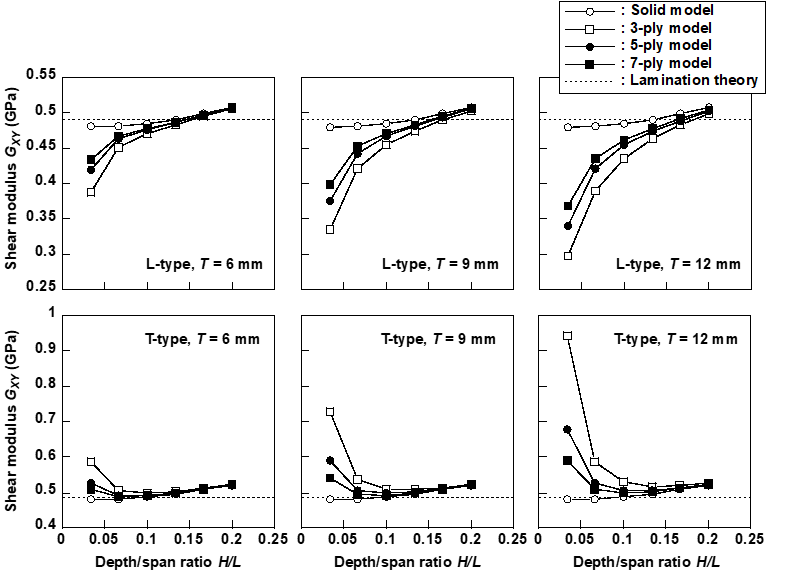Fig. 4. Dependence of the in-plane shear modulus (GXY) on the depth/span ratio (H/L) corresponding to the ply numbers and total thickness of the plywood model obtained by FEA

For the L-type model, the GXY value tended to decrease as the H/L ratio decreased. This decreasing tendency is commonly observed in the GXYH/L relationship obtained from modal analysis as shown in a previous study (Yoshihara 2012). Similarly, the GXY value of the T-type model tended to decrease as the H/L ratio decreased, when H/L was larger than 0.1. However, this value increased markedly as the H/L ratio decreased when the H/L was smaller than 0.1. This trend is markedly different than that observed in the modal analysis of the T-type model (Yoshihara 2012). Nevertheless, these trends were pronounced as the ply number decreased and the thickness of the plywood (T) increased, which corresponded to the breadth of the FE model. These pronounced tendencies are commonly observed in the results of modal analysis (Yoshihara 2012).

According to beam theory, in-plane shear stress corresponding to the distance from the neutral axis at the mid-span can be derived as follows:(5)

where Y is the distance from the neutral axis in the depth direction (−H/2 ≤ Y ≤ H/2). Equation (5) suggests that the 4XYTH/3P value should be equal to 1 − 4(Y/H)2 when the load-deformation behavior obtained from the FEA perfectly coincides with that derived by beam theory. Figure 5 shows the relationship between the Y/H and 4XYTH/3P values obtained from the FEAs. When the ply number and specimen depth decrease, the shear stress distribution obtained from the FEA deviates significantly from that derived by beam theory. Because of the deviation, there is a concern that the in-plane shear modulus cannot be obtained accurately using Eq. (1), which is based on beam theory.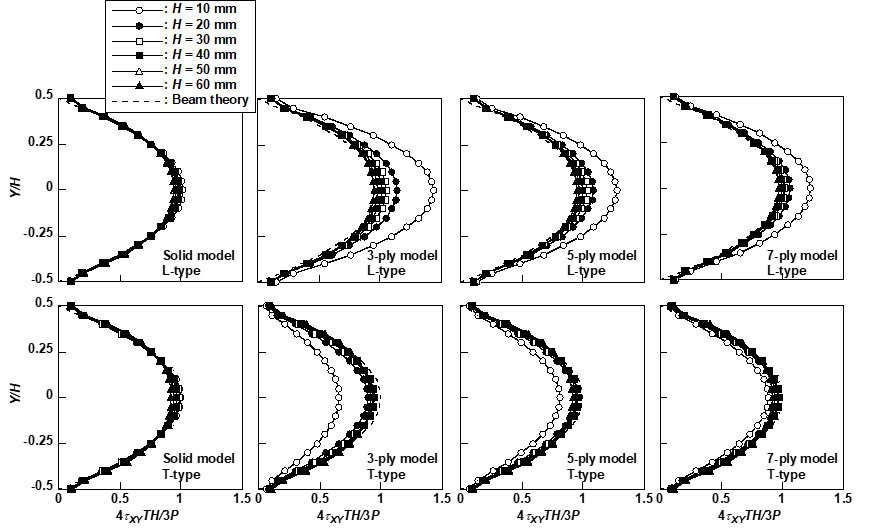Fig. 5. Distribution of the normalized shear stress (4XYTH/3P) along the depth direction (Y-axis) at the mid-span of the surface veneer obtained by FEA. Breadth of the model T = 9 mm

AFPB Tests

Figure 6 presents a comparison of the GXY values determined from the AFPB and FV tests. Similar to the FEA results, the GXY values obtained from the L-type specimen decreased with decreasing H/L ratio, whereas the values obtained from the T-type specimen decreased when the H/L ratio was larger than 0.1 and increased markedly when the H/L ratio was smaller than 0.1. In the FV test conducted in a previous study (Yoshihara 2012), the effect of the specimen configuration was reduced when the H/L ratio was smaller than 0.1. Therefore, the GXY value could be measured accurately by the FV test using the specimen with the initial configuration. In contrast, the dependence of the GXY value on the H/L ratio obtained from the AFPB test was more significant than that obtained from the FV test. The dependence of the GXY value on the H/L ratio obtained from the AFPB test may be because of the restriction of deformation between the veneers, which induces the deviation of the load-deformation behavior of the specimen, from beam theory as described above. Therefore, the GXY values obtained from the AFPB tests are often different from those obtained from the FV test conducted here, which is thought to be accurate enough. From the experimental results, however, the source of the dependence could not be revealed because the detailed distribution of the deformation was not observed in this study. It may be revealed when conducting full-field observation such as digital image correlation (DIC) (Murata et al. 2011).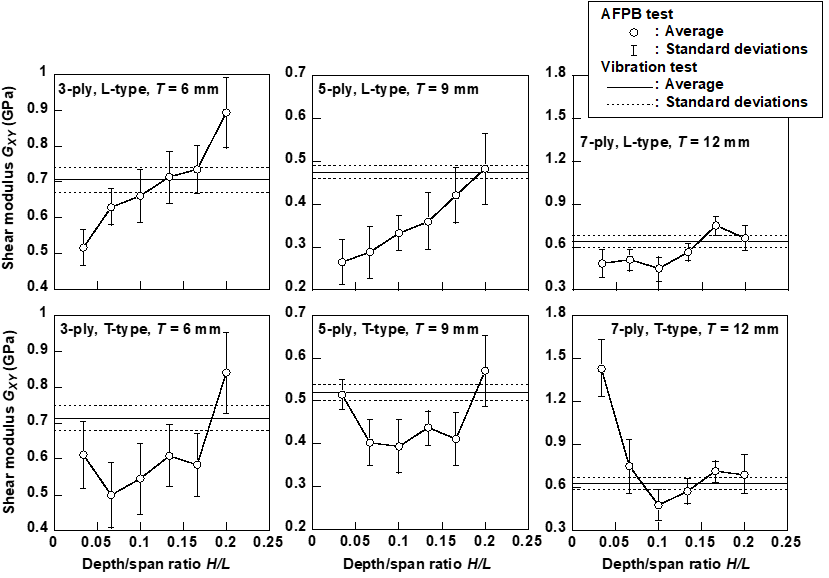Fig. 6. Shear modulus (GXY) obtained using the asymmetric four-point bending (AFPB) and flexural vibration (FV) tests. The FV test was conducted using the specimen with the initial dimensions.

Table 5 represents the statistical analysis results of the difference between the means of the GXY values obtained from the AFPB and FV tests. The statistical analyses showed that the GXYvalues obtained from the AFPB test were similar to those obtained from the FV tests, except for a few results when the H/L ratio was larger than 0.133. In this range of H/L ratios, however, the GXY values still varied when varying the H/L ratio. In the results obtained from the FV tests conducted using various specimen configure-tions, the GXY value could be obtained accurately by reducing the effect of specimen configuration by making the depth/length ratio larger than 0.1 (Yoshihara 2012). In this depth/length ratio range, the GXY value obtained from the FV test could be regarded as constant and independent of the depth/length ratio.

Table 5. Statistical Analysis Results of the Difference between the Means of In-plane Shear Moduli Obtained from the AFPB and FV Tests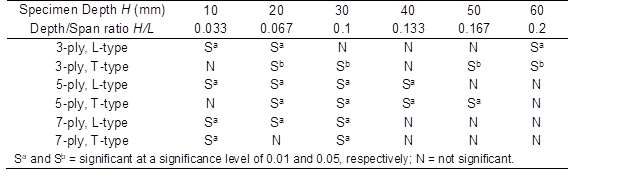From the results obtained in this study, it is clear that the depth/span ratio should be restricted to enable accurate measurement of the in-plane shear modulus in an AFPB test. Comparing the results obtained from the AFPB and FV tests, the FV test was superior to the AFPB test for accurately measuring the in-plane shear modulus of plywood for a wide range of specimen configurations, although multiple vibration tests should be conducted to determine the in-plane shear modulus.

CONCLUSIONS

1. The experimental and numerical results suggested that the in-plane shear modulus of an L-type specimen tended to decrease as the depth/span ratio decreased. This tendency was similar to that observed for the FV test conducted in a previous study (Yoshihara 2012).
2. The in-plane shear modulus of a T-type specimen tended to decrease as the depth/span ratio decreased when the depth/span ratio was larger than 0.1. When the depth/span ratio was smaller than 0.1, however, this value markedly increased as the depth/span ratio decreased. This tendency was significantly different from that observed in the FV test conducted in a previous study (Yoshihara 2012).
3. The dependence of the in-plane shear modulus on the specimen configuration was more significant in the AFPB test than in the FV test. To measure the in-plane shear modulus of plywood accurately, the FV test is therefore more recommended than the AFPB test.

ACKNOWLEDGMENTS

A part of this work was supported by a Grant-in-Aid for Scientific Research (C) (No. 24580246) of the Japan Society for the Promotion of Science (JSPS).

REFERENCES CITED

Adams, D. F., Carlsson, L. A., and Pipes, R. B. (2003). Experimental Characterization of Advanced Composite Materials, 3rd Edition, CRC Press, Boca Raton, FL.

Ansys (2009). ANSYS Release 12.0, Ansys Inc., Canonsburg, PA.

Chida, T., Sasaki, T., Yamauchi, H., Okazaki, Y., Kawai, Y., and Iijima, Y. (2012). “A proposed standard test method for shear failure and estimation of shear strength of Japanese cedar (1) Shear failure test of Japanese cedar laminates using wood material as stiffener and finite element analysis, and estimation of shear modulus,” Mokuzai Gakkaishi 58(5), 260-270.

Forestry and Forest Products Research Institute (2004). Wood Industry Handbook, Maruzen, Tokyo, Japan.

Hearmon, R. F. S. (1948). Elasticity of Wood and Plywood, HM Stationary Office, London, UK.

Mathematica 6 (2007). Wolfram Research, Champaign, IL.

Morita, H., Fujimoto, Y., Komatsu, K., and Murase, Y. (2006). “Development of a shear testing method for full-sized structural lumber,” Mokuzai Gakkaishi 52(6), 376-382.

Murata, K., Nagai, H., and Nakano, T. (2011). “Estimation of width of fracture process zone in spruce wood by radial tensile test,” Mech. Mater. 43(7), 389-396.

Nairn, J. (2007). “Numerical implementation of imperfect interfaces,” Comput. Mater. Sci. 40(4), 525-536.

Takami, I. (1964). “Poisson’s ratio of plywood,” Bull. Gov. Forest Exp. Sta. 49, 133-174.

Yoshihara, H., and Suzuki, A. (2005). “Shear stress/shear strain relation of wood obtained by asymmetric four-point bending test of side-tapered specimen,” J. Test. Eval. 33(1), 55-60.

Yoshihara, H. (2006). “Characterization of shear properties of wood and wood-based materials,” J. Soc. Mater. Sci. Jpn. 55(4), 349-355.

Yoshihara, H. (2009a). “Edgewise shear modulus of plywood measured by square-plate twist and beam flexure methods,” Construct. Build. Mater. 23(12), 3537-3345.

Yoshihara, H. (2009b). “Shear properties of wood measured by the asymmetric four-point bending test of notched specimen,” Holzforschung 63(2), 211-216.

Yoshihara, H. (2009c). “Poisson’s ratio of plywood measured by tension test,” Holzforschung 63(5), 603-608.

Yoshihara, H. (2010). “Measurement of the in-plane shear modulus of plywood by tension test of  off-axis specimen,” Trans. Jpn. Soc. Mech. Eng. A 77(776), 670-678.

Yoshihara, H. (2012). “Influence of the specimen depth to length ratio and lamination construction on Young’s modulus and in-plane shear modulus of plywood measured by flexural vibration,” BioResouces 7(1), 1337-1351.

Yoshihara, H. (2013a). “Flatwise Young’s modulus and flatwise shear modulus of plywood measured by flexural vibration test,” Holzforschung DOI: 10.1515/hf-2012-0174.

Yoshihara, H. (2013b). “Comparison of results obtained by static 3- and 4-point bending and flexural vibration tests on solid wood, MDF, and 5-plywood,” Holzforschung (in press).

Article submitted: April 3, 2013; Peer review completed: May 21, 2013; Revised version received: May 26, 2013; Accepted: May 30, 2013; Published: June 3, 2013.